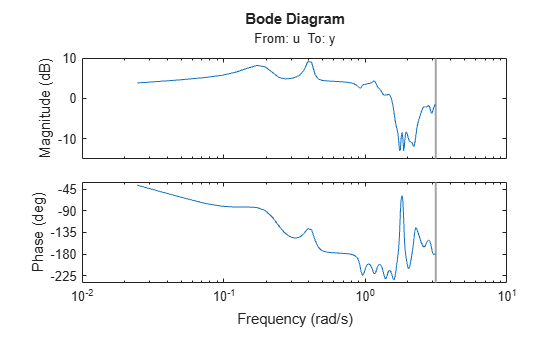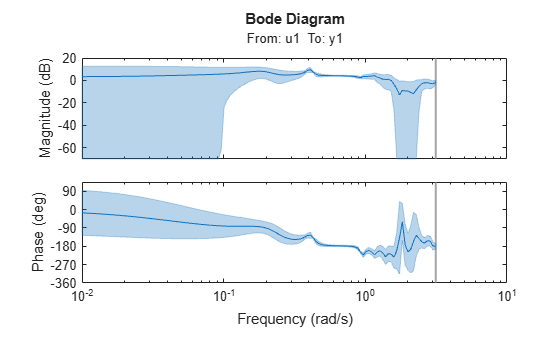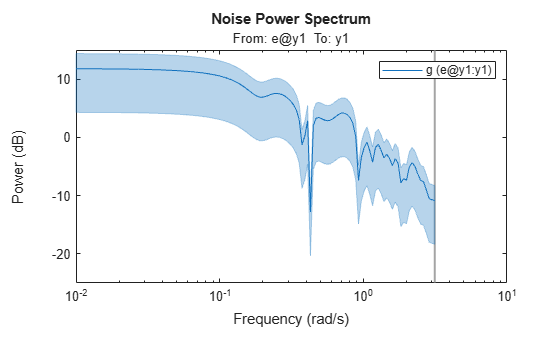# spa

Estimate frequency response with fixed frequency resolution using spectral analysis

## Syntax

``G = spa(data)``
``G = spa(data,winSize,freq)``
``G = spa(data,winSize,freq,maxSize)``

## Description

example

````G = spa(data)` estimates the frequency response, along with uncertainty, and the noise spectrum from time- or frequency-domain data `data`. If `data` is a time series, `spa`(`data)` returns the output power spectrum along with uncertainty. `spa` computes the spectra at 128 equally spaced frequency values between 0 (excluded) and π, using a Hann window.```

example

````G = spa(data,winSize,freq)` estimates the frequency response at the frequencies contained in `freq`, using a Hann window with size `winSize`.```
````G = spa(data,winSize,freq,maxSize)` splits the input/output data into segments, each segment containing fewer than `maxSize` elements. Use this syntax to improve computational performance.```

## Examples

collapse all

Estimate the frequency response for the input/output data in the `iddata` object `z3`. Use the default fixed resolution of 128 equally spaced logarithmic frequency values between 0 (excluded) and $\pi$.

```load iddata3 z3; g = spa(z3); bode(g)```Generate the logarithmically spaced vector `f`.

`f = logspace(-2,pi,128);`

Estimate the frequency response for the input/output data `z3`. Specify the window size as `[]` to obtain the default lag window size.

```load iddata3 z3; g = spa(z3,[],f);```

Plot the Bode response and disturbance spectrum with a confidence interval of 3 standard deviations.

```h = bodeplot(g); showConfidence(h,3)``````figure h = spectrumplot(g); showConfidence(h,3)```## Input Arguments

collapse all

Input/output data, specified as an `iddata` object or an `idfrd` object that can contain complex values. `data` can also contain time series data with only output.

Hann window size, also known as lag size, specified as a scalar integer. By default, the function sets the window size to `min(length(data)/10,30)`.

Frequencies at which to estimate spectral response, specified as a row vector in units of rad/`TimeUnit`, where `TimeUnit` refers to the `TimeUnit` property of `data`. By default, the function sets `freq` to a vector of 128 values in the range (0,π], evenly spaced logarithmically. For discrete-time models, set `freq` within the Nyquist frequency bound.

Maximum size of segments within `data`, specified as a positive integer. If you omit this argument, the function performs estimation using the full data set in `data` rather than segmenting the data.

## Output Arguments

collapse all

Frequency response with uncertainty and noise spectrum, specified as an `idfrd` object. For time series data, `G` is the estimated spectrum and standard deviation.

Information about the estimation results and options used is stored in the `Report` property of the model. `Report` has the following fields.

Report FieldDescription
`Status`

Summary of the model status, which indicates whether the model was created by construction or obtained by estimation.

`Method`

Estimation command used.

`windowSize`

Size of the Hann window.

`DataUsed`

Attributes of the data used for estimation. Structure with the following fields.

FieldDescription
`Name`

Name of the data set.

`Type`

Data type.

`Length`

Number of data samples.

`Ts`

Sample time. This is equivalent to `data.Ts`.

`InterSample`

Input intersample behavior. One of the following values:

• `'zoh'` — Zero-order hold maintains a piecewise-constant input signal between samples.

• `'foh'` — First-order hold maintains a piecewise-linear input signal between samples.

• `'bl'` — Band-limited behavior specifies that the continuous-time input signal has zero power above the Nyquist frequency.

The value of `InterSample` has no effect on estimation results for discrete-time models.

`InputOffset`

Offset removed from time-domain input data during estimation.

`OutputOffset`

Offset removed from time-domain output data during estimation.

collapse all

### Frequency Response Function

A frequency response function describes the steady-state response of a system to sinusoidal inputs. For a linear system, a sinusoidal input of a specific frequency results in an output that is also a sinusoid with the same frequency, but with a different amplitude and phase. The frequency response function describes the amplitude change and phase shift as a function of frequency.

To better understand the frequency response function, consider the following description of a linear dynamic system:

`$y\left(t\right)=G\left(q\right)u\left(t\right)+v\left(t\right)$`

Here, u(t) and y(t) are the input and output signals, respectively. G(q) is called the transfer function of the system—it captures the system dynamics that take the input to the output. The notation G(q)u(t) represents the following operation:

`$G\left(q\right)u\left(t\right)=\sum _{k=1}^{\infty }g\left(k\right)u\left(t-k\right)$`

q is the shift operator, defined by the following equation:

G(q) is the frequency-response function when it is evaluated on the unit circle, G(q=e).

Together, G(q=e) and the output noise spectrum ${\stackrel{^}{\Phi }}_{v}\left(\omega \right)$ compose the frequency-domain description of the system.

The frequency-response function estimated using the Blackman-Tukey approach is given by the following equation:

`${\stackrel{^}{G}}_{N}\left({e}^{i\omega }\right)=\frac{{\stackrel{^}{\Phi }}_{yu}\left(\omega \right)}{{\stackrel{^}{\Phi }}_{u}\left(\omega \right)}$`

In this case, ^ represents approximate quantities. For a derivation of this equation, see the chapter on nonparametric time- and frequency-domain methods in .

### Output Noise Spectrum

The output noise spectrum (spectrum of v(t)) is given by the following equation:

`${\stackrel{^}{\Phi }}_{v}\left(\omega \right)={\stackrel{^}{\Phi }}_{y}\left(\omega \right)-\frac{{|{\stackrel{^}{\Phi }}_{yu}\left(\omega \right)|}^{2}}{{\stackrel{^}{\Phi }}_{u}\left(\omega \right)}$`

This equation for the noise spectrum is derived by assuming that the linear relationship $y\left(t\right)=G\left(q\right)u\left(t\right)+v\left(t\right)$ holds, that u(t) is independent of v(t), and that the following relationships between the spectra hold:

`${\Phi }_{y}\left(\omega \right)={|G\left({e}^{i\omega }\right)|}^{2}{\Phi }_{u}\left(\omega \right)+{\Phi }_{v}\left(\omega \right)$`
`${\Phi }_{yu}\left(\omega \right)=G\left({e}^{i\omega }\right){\Phi }_{u}\left(\omega \right)$`

Here, the noise spectrum is given by the following equation:

`${\Phi }_{v}\left(\omega \right)\equiv \sum _{\tau =-\infty }^{\infty }{R}_{v}\left(\tau \right){e}^{-iw\tau }$`

${\stackrel{^}{\Phi }}_{yu}\left(\omega \right)$ is the output-input cross-spectrum and ${\stackrel{^}{\Phi }}_{u}\left(\omega \right)$ is the input spectrum.

Alternatively, the disturbance v(t) can be described as filtered white noise:

`$v\left(t\right)=H\left(q\right)e\left(t\right)$`

Here, e(t) is the white noise with variance $\lambda$ and the noise power spectrum is given by the following equation:

`${\Phi }_{v}\left(\omega \right)=\lambda {|H\left({e}^{i\omega }\right)|}^{2}$`

## Algorithms

`spa` applies the Blackman-Tukey spectral analysis method by following these steps:

1. Compute the covariances and cross-covariance from u(t) and y(t):

`$\begin{array}{l}{\stackrel{^}{R}}_{y}\left(\tau \right)=\frac{1}{N}\sum _{t=1}^{N}y\left(t+\tau \right)y\left(t\right)\\ {\stackrel{^}{R}}_{u}\left(\tau \right)=\frac{1}{N}\sum _{t=1}^{N}u\left(t+\tau \right)u\left(t\right)\\ {\stackrel{^}{R}}_{yu}\left(\tau \right)=\frac{1}{N}\sum _{t=1}^{N}y\left(t+\tau \right)u\left(t\right)\end{array}$`
2. Compute the Fourier transforms of the covariances and the cross-covariance:

`$\begin{array}{l}{\stackrel{^}{\Phi }}_{y}\left(\omega \right)=\sum _{\tau =-M}^{M}{\stackrel{^}{R}}_{y}\left(\tau \right){W}_{M}\left(\tau \right){e}^{-i\omega \tau }\\ {\stackrel{^}{\Phi }}_{u}\left(\omega \right)=\sum _{\tau =-M}^{M}{\stackrel{^}{R}}_{u}\left(\tau \right){W}_{M}\left(\tau \right){e}^{-i\omega \tau }\\ {\stackrel{^}{\Phi }}_{yu}\left(\omega \right)=\sum _{\tau =-M}^{M}{\stackrel{^}{R}}_{yu}\left(\tau \right){W}_{M}\left(\tau \right){e}^{-i\omega \tau }\end{array}$`

where ${W}_{M}\left(\tau \right)$ is the Hann window with a width (lag size) of M. You can specify M to control the frequency resolution of the estimate, which is approximately equal to 2π/M rad/sample time.

By default, this operation uses 128 equally spaced frequency values between 0 (excluded) and π, where `w` = `[1:128]/128*pi/Ts` and `Ts` is the sample time of that data set. The default lag size of the Hann window is ```M = min(length(data)/10,30)```. For default frequencies, the operation uses fast Fourier transforms (FFT), which are more efficient than for user-defined frequencies.

Note

`M =` γ is in Table 6.1 of . Standard deviations are on pages 184 and 188 in .

3. Compute the frequency-response function ${\stackrel{^}{G}}_{N}\left({e}^{i\omega }\right)$ and the output noise spectrum ${\stackrel{^}{\Phi }}_{v}\left(\omega \right)$.

`${\stackrel{^}{G}}_{N}\left({e}^{i\omega }\right)=\frac{{\stackrel{^}{\Phi }}_{yu}\left(\omega \right)}{{\stackrel{^}{\Phi }}_{u}\left(\omega \right)}$`
`${\Phi }_{v}\left(\omega \right)\equiv \sum _{\tau =-\infty }^{\infty }{R}_{v}\left(\tau \right){e}^{-iw\tau }$`

`spectrum` is the spectrum matrix for both the output and the input channels. That is, if `z = [data.OutputData`, `data.InputData]`, `spectrum` contains as spectrum data the matrix-valued power spectrum of `z`.

`$S=\sum _{m=-M}^{M}Ez\left(t+m\right)z{\left(t\right)}^{\prime }{W}_{M}\left({T}_{s}\right)\mathrm{exp}\left(-i\omega m\right)$`

Here, `'` is a complex-conjugate transpose.

 Ljung, Lennart. System Identification: Theory for the User. 2nd ed. Prentice Hall Information and System Sciences Series. Upper Saddle River, NJ: Prentice Hall PTR, 1999.